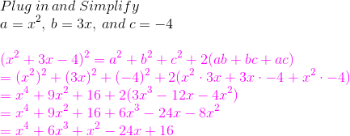# How to Square a Trinomial

An error occurred trying to load this video.

Try refreshing the page, or contact customer support.

Coming up next: Combining Like Terms with the Distributive Property

### You're on a roll. Keep up the good work!

Replay
Your next lesson will play in 10 seconds
• 0:03 Trinomial Expressions
• 0:17 Squaring a Trinomial
• 2:34 Origins of the Formula

Want to watch this again later?

Timeline
Autoplay
Autoplay
Speed

#### Recommended Lessons and Courses for You

Lesson Transcript
Instructor: Laura Pennington

Laura has taught collegiate mathematics and holds a master's degree in pure mathematics.

Trinomials are polynomials with three terms. In this lesson, we'll see how to square a trinomial using a formula and learn how the formula is derived - which gives us another way to square a trinomial!

## Trinomial Expressions

In mathematics, a trinomial is an algebraic expression that has three terms and takes on the form a + b + c. The terms a, b, and c can be numbers, variables, or some combination of the two.

## Squaring a Trinomial

When we square a trinomial, we square it the same way we would any ordinary number or variable: we multiply it by itself.

(a + b + c)2 = (a + b + c)(a + b + c)

Now, before we go any further, it's important to note that (a + b + c)2a2 + b2 + c2 - an extremely common error.

Okay, now that we've established that, let's look at how to multiply a trinomial by itself. To square a trinomial, we use the following formula:

(a + b + c)2 = a2 + b2 + c2 + 2(ab + bc + ac)

To square a trinomial, all we have to do is follow these two steps:

1. Identify a as the first term in the trinomial, b as the second term, and c as the third term
2. Plug a, b, and c into the formula

For example, suppose we wanted to square the trinomial x2 + 3x - 4. First, we'll identify the first term as a = x2, the second term as b = 3x, and the third term as c = -4. Now, we just plug the values into the formula and simplify!As we said earlier, it's just a matter of multiplying the terms and adding them up.

## Origins of the Formula

So, where did this formula come from? Let's take a look at how to derive this formula and, in the process, you may find that you like this pattern for squaring a trinomial better than using the formula itself!

As we said earlier, when we square a trinomial, we are multiplying it by itself:

(a + b + c)2 = (a + b + c)(a + b + c)

Now, to multiply any two algebraic expressions with multiple terms (like a trinomial) together, we follow this pattern:

To unlock this lesson you must be a Study.com Member.

### Register to view this lesson

Are you a student or a teacher?

### Unlock Your Education

#### See for yourself why 30 million people use Study.com

##### Become a Study.com member and start learning now.
Back
What teachers are saying about Study.com

### Earning College Credit

Did you know… We have over 160 college courses that prepare you to earn credit by exam that is accepted by over 1,500 colleges and universities. You can test out of the first two years of college and save thousands off your degree. Anyone can earn credit-by-exam regardless of age or education level.